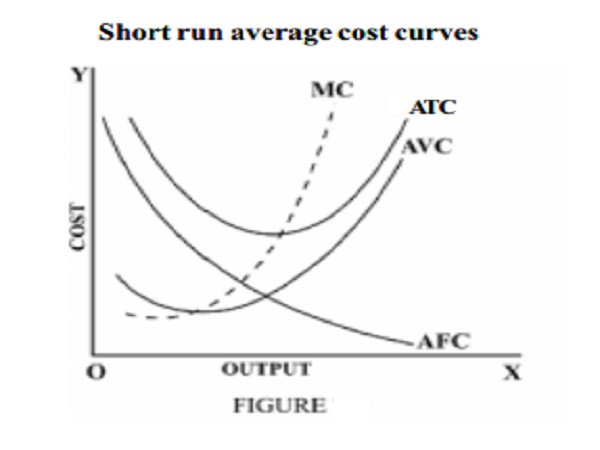Home | | Economics | | Economics | Short run average cost curves - Average Fixed, Average variable and Average Total Cost

# Short run average cost curves - Average Fixed, Average variable and Average Total CostThe average fixed cost is the fixed cost per unit of output. It is obtained by dividing the total fixed cost by the number of units of the commodity produced.

Short run average cost curves

Average Fixed Cost (AFC)

The average fixed cost is the fixed cost per unit of output. It is obtained by dividing the total fixed cost by the number of units of the commodity produced.

Symbolically        AFC = TFC / Q

Where   AFC = Average fixed Cost TFC = Total Fixed cost

Q   = number of units of output produced

Suppose for a firm the total fixed cost is Rs 2000 when output is 100 units, AFC will be Rs 2000/100 = Rs 20 and when output is 200 units, AFC will be Rs 2000/200 = Rs10/- Since total fixed cost is a constant quantity, average fixed cost will steadily fall as output increases; when output becomes very large, average fixed cost approaches zero.

Average Variable cost (AVC): Average variable cost is the variable cost per unit of output. It is the total variable cost divided by the number of units of output produced.

AVC = TVC / Q

Where AVC = Average Variable Cost

TVC       = Total Variable Cost

Q            = number of units of output produced

Average variable cost curve is 'U' Shaped. As the output increases, the AVC will fall upto normal capacity output due to the operation of increasing returns. But beyond the normal capacity output, the AVC will rise due to the operation of diminishing returns.

Average Total Cost or Average Cost : Average total cost is simply called average cost which is the total cost divided by the number of units of output produced.

AC = TC / Q where AC = Average Cost TC = Total Cost

Q = number of units of output produced

Average cost is the sum of average fixed cost and average variable cost. i.e. AC = AFC+AVC

Table Calculation of Average Fixed, Average variable and Average Total Cost

Units of      TFC  TVC TC    AFC  AVC AC

output                           2 � 1  3 � 1  5 + 6

1        2        3        4        5        6        7

0        120    0        120    -        0        -

1        120    100    220    120    100    220

2        120    160    280    60      80      140

3        120    210    330    40      70      110

4        120    240    360    30      60      90

5        120    400    520    24      80      104

6        120    540    660    20      90      110

7        120    700    820    17.14 100    117.14

8        120    880    1000  15      110    125The average cost is also known as the unit cost since it is the cost per unit of output produced. The following figure shows the shape of AFC, AVC and ATC in the short period.

From the figure , it can be understood that the behaviour of the average total cost curve depends on the behaviour of AFC and AVC curves. In the beginning, both AFC and AVC fall. So ATC curve falls. When AVC curve begins rising, AFC curve falls steeply ie fall in AFC is more than the rise in AVC. So ATC curve continues to fall. But as output increases further, there is a sharp increase in AVC, which is more than the fall in AFC. Hence ATC curve rises after a point. The ATC curve like AVC curve falls first, reaches the minimum value and then rises. Hence it has taken a U shape.

Study Material, Lecturing Notes, Assignment, Reference, Wiki description explanation, brief detail

Related Topics